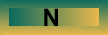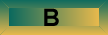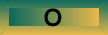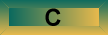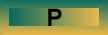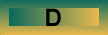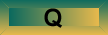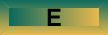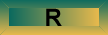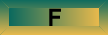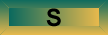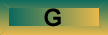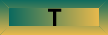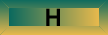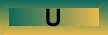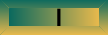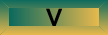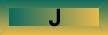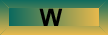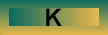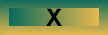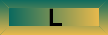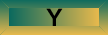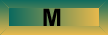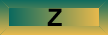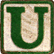Universal Function Approximator A universal function approximator is a system that, given a set of predictor variables, can output an accurate estimate of some predicted variable.  That is, it can compute the value f(x) on the basis of some x, or some set of x’s.  For example, Churchland’s crab computes the angle of its arm’s elbow on the basis of the angles of the two eyes that are looking at a target.  For a function approximator to be universal, it must be able to compute the value of any function to an arbitrary degree of precision, at least in some range of the function.  This concept is important because it has been shown that if one permits enough hidden units, artificial neural networks have the capability to be universal function approximators (Hartman et al., 1989; Hornik et al., 1989), and are therefore sufficiently powerful to be of interest to cognitive science (e.g., to serve as plausible alternatives to universal Turing machines). References: Hartman, E., Keeler, J. D., & Kowalski, J. M. (1989). Layered neural networks with Gaussian hidden units as universal approximation. Neural computation, 2, 210-215. Hornik, M., Stinchcombe, M., & White, H. (1989). Multilayer feedforward networks are universal approximators. Neural Networks, 2, 359-366. (Added November 2009)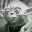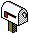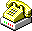(780)-492-5175
 WWW www.bcp.psych.ualberta.ca